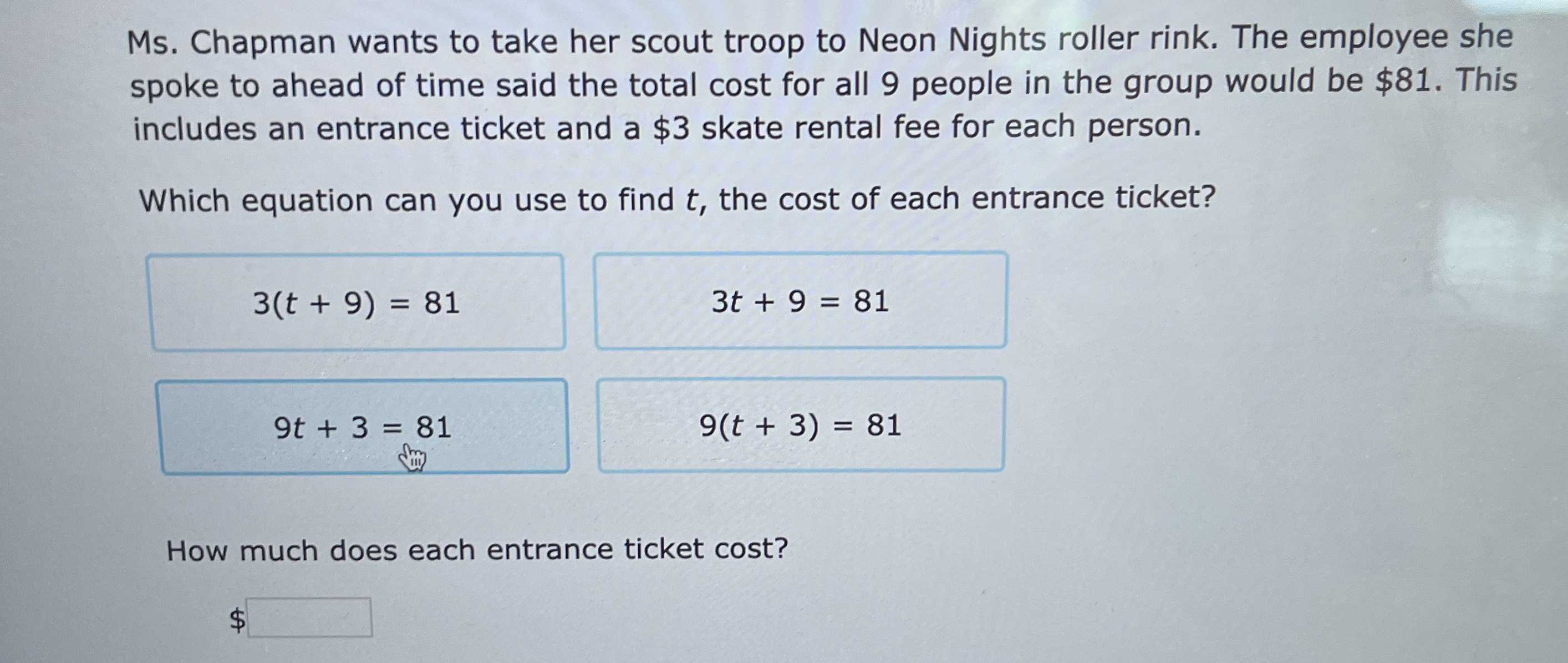### Still have math questions?

Algebra
QuestionMs. Chapman wants to take her scout troop to Neon Nights roller rink. The employee she spoke to ahead of time said the total cost for all $$9$$ people in the group would be $$\ 81$$ . This includes an entrance ticket and a $$\ 3$$ skate rental fee for each person. Which equation can you use to find $$t$$ , the cost of each entrance ticket?

$$3 ( t + 9 ) = 81$$                        $$3 t + 9 = 81$$

$$9 t + 3 = 81$$                   $$9 ( t ) = 81$$

How much does each entrance ticket cost?

\$